Preferred Citation: Darrigol, Olivier. From c-Numbers to q-Numbers: The Classical Analogy in the History of Quantum Theory. Berkeley:  University of California Press,  1992. http://ark.cdlib.org/ark:/13030/ft4t1nb2gv/Chapter IV The Infrared Challenge## Chapter IV The Infrared Challenge

The pretense of Planck's theory of radiation to determine the blackbody law was soon contradicted by Berlin's best spectroscopists. In 1899 Paschen, Lummer, and Pringsheim observed violations of Wien's blackbody law in the infrared part of the spectrum. Even though he did not immediately take this result as irreproachable, Planck came to recognize that the proof of the electromagnetic H -theorem was compatible with an infinite number of choices for S, the resonator entropy. As will presently be seen, the only necessary restriction on this choice was that the second derivative of S be negative: d 2S/dU2 < 0. Subsequently, Planck found a physical meaning for this derivative and used it to justify the choice of S(U ) that led to Wien's law.

### The Second Derivative of the Resonator Entropy

The meaning of d2 S/dU2 derives from Planck's following consideration. Having already quickly increased (or decreased) the energy of a resonator initially in equilibrium with thermal radiation by an amount d U, one allows this energy to relax by dU (with dU << d U) toward its equilibrium

 This has to do with the fact that ideal resonators cannot change at all the spectrum of cavity radiation (see n. 49). An infinite number of radiation spectra are compatible with Planck's system, as implied by the infinite number of possible choices for the resonator entropy.

 Planck 1900b. On infrared blackbody measurements see Jammer 1966,16-17; Mehra and Rechenberg 1982a, 39-43.

58

value. The derivative d 2S/du2 is then proportional to the total entropy change, dST , occurring during the relaxation process. More exactly, Planck proved that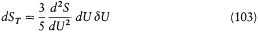in the following manner.

During the increment of U by d U the beam intensity balance DI (as defined on p. 46) goes from zero (equilibrium) to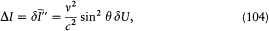since, according to (85),is the only intensity that depends on the energy of the resonator. Accordingly, at the second order of approximation the entropy-intensity balance goes from zero toIn this development the index zero refers to the original state of equilibrium, for which all I 's are equal to v2U/c2 . Now let a time dt elapse after the excitation of the resonator. The corresponding relaxation of the resonator energy, dU , is obtained by substituting the above DI into the equation (86) of energy conservation:In the same time interval the total entropy variation (from (90)) is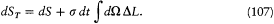Inserting the development (105) in the latter equation and using again the equation (86) of energy conservation yields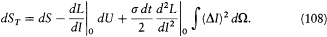The first term can be rewritten asPlanck 1900b, PAV 1:679.

59

and the integral in the last term can be evaluated using (104), (106), and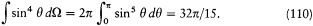The resulting expression for dS T reads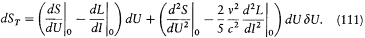So that the system may evolve toward equilibrium, dUd U must be negative; but the individual signs of dU and d U are not fixed. Consequently, the sign of the above expression of dST is definite if and only if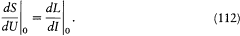This identity must hold for all values of I and U related through I = Uv2/c2 , which implies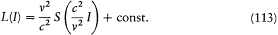and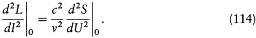Taking into account the latter remarks, the expression (111) collapses into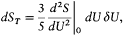which was to be proved.

This calculation gave two necessary conditions for entropy increase: the form (113) for the function L (I ) corresponding to the function S (U ), and the convexity condition for the latter function, d2/dU 2 < 0, which provides dS T < 0 (since dUd U < 0). As Planck now noticed, these conditions were also sufficient for entropy increase. Indeed, the proof of the electro-magnetic H -theorem earlier given may be adapted in the following way.

Starting from the equations (86) and (87) for energy and entropy balance, one can use the first condition (113) to derive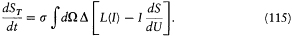Then, the two conditions together imply that L(I) - IdS/dU (at a constant U ) is a convex function of I , with an absolute maximum for I = v2U/c 2 These properties are the only ones necessary to the rest of the

60

proof of entropy increase. That Planck originally believed the form S = - (U/f ) In (U/g ) to be the only one possible may be explained by the intricacy of his own proof of the electromagnetic H -theorem, which did not exploit the convexity of S (U ).

Once aware of the physical meaning of d2S/dU 2 , Planck considered a set of n resonators (separated from one another by large distances) immersed in the same thermal radiation and submitted to the same perturbation d U. The total entropy variation during a common relaxation dU of the energy of these resonators is n dST . Planck then equated this variation with the one obtained in the case of a single resonator with the initial energy nU submitted to the perturbation nd U and relaxing by n dU , which gives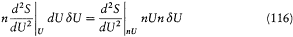or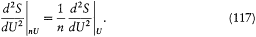This property implies the form d2S/dU 2= -a /U, which integrates into Planck's original formula S = - (U/f) ln (U/g ) and therefore implies Wien's law.

Planck's colleagues quickly perceived the mistake in the above reasoning: a single resonator with the energy nU is not in equilibrium with the same thermal radiation as the n resonators with the energy U , so that there is no reason to equate the relaxation rates in the two cases. Moreover, by the summer of 1900 the violations of Wien's law in the infrared range had become incontestable. On 19 October, Planck acknowledged his mistake at the Berlin Academy. His methods were really unable to provide a precise form of the resonator entropy and were in fact compatible with an infinity of different equilibrium distributions. He nevertheless "guessed" a new blackbody law, starting from an expression for the second derivative of the resonator entropy S (U ).

 Of course Planck's presentation of this argument does not necessarily reflect the chronological order of his considerations. Perhaps he first noticed the extreme simplicity of the second derivative of the entropy corresponding to Wien's law and then sought a physical interpretation of this derivative that would justify the property of homogeneity.

 Planck 1900c. See Kuhn 1978, 96-97.

61

This expression, he argued, had to be negative in order not to contradict the entropy law; it had to give back the form - 1/a U leading to Wien's law for small values of U (that is to say for large frequencies); and, for the sake of simplicity, it had to be easily integrable. Planck therefore conjectured the formwhose integration gives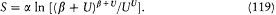This formula, once combined with dS/dU = 1/T , Wien's displacement law, and the fundamental equation uv = (8 p v2/c3 )U , leads to "Planck's law":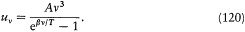Within a few days blackbody spectroscopists could verify how excellently this law fitted their experimental data.

In the same communication Planck noted that the form of S (U ) was logarithmic, "as suggested by probability calculus." Presumably he was already hoping for a deeper justification of this law based on Boltzmann's relation between entropy and combinatorial probability. He was here confronted with a situation different from that occurring in Boltzmann's gas theory. In the gas case the Boltzmann equation provided by itself the equilibrium distribution, and the method of complexions gave hardly more than "an illustration of the mathematical meaning of the quantity H" of the H -theorem, as Boltzmann wrote in his Gastheorie . Instead, in Planck's radiation theory the electromagnetic H -theorem had nothing to say about the equilibrium spectrum, while the method of complexions seemed to offer a new hope of a derivation of this spectrum.

This is why Planck decided to turn to the combinatorial method. However, he consistently rejected the probabilistic context of Boltzmann's original considerations. One could well call a certain mathematical function of the state of a system a "probability" without having to consider the increase of this function in time as a matter of probability. Such was Planck's

 Planck 1900c, PAV 1:689; Boltzmann 1896, trans., 55. In Boltzmann's H -theorem the temperature is unambiguously defined through the average kinetic energy corresponding to the distribution f (v ). In the electromagnetic H -theorem the only available definition of temperature is through the relation dS/dU = 1/T .

62

opinion, as already expressed in a letter to Graetz of 1897:

Probability calculus can serve, if nothing is known in advance, to determine a state [of equilibrium] as the most probable one. But it cannot serve, if an improbable state is given, to compute the following state. That is determined not by probability but by mechanics.

The relation between entropy and probability had been introduced by Boltzmann in 1877 in the context earlier described (see pp. 16-17). It is now time to specify the mathematical content of these considerations, on which Planck's success very much depended.

### Boltzmann's Combinatorics

The basic object of Boltzmann's combinatorics of 1877 is a perfect gas of point molecules, a microstate of which is characterized by the set of molecule velocities and positions. In a first simplifying step, Boltzmann considers only the kinetic energies of the molecules and tries to define the probability of an energy partition (Energieverteilung ), that is, of a distribution of a given total energy E over the molecules. Since energy is a continuous variable, there is no obvious definition of such a probability.

As in his combinatorial considerations of 1868, Boltzmann starts with a "fiction" wherein molecules can take only discrete energy values 0, e , 2e , . . ., i e , . . . . Then, if molecules are labeled by the index (a = 1, 2, . . ., N ), a microstate of the system, or "complexion," is defined by attributing to each molecule a given energy: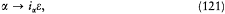where iae is an integral multiple of e . An "energy partition" is given by a sequence of integers N0 , N 1 , . . ., Ni , . . ., where Ni is the number of molecules carrying the energy i e . To a given partition corresponddifferent Komplexionen . Boltzmann calls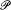the "permutability," since it is equal to the number of permutations of the N molecules that transfer at least one molecule from one discrete energy value to another: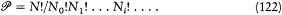The probability W of a partition (N0 , N 1 , . . ., Ni , . . .) is obtained through division of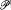by the normalization factor; Boltzmann gave an explicit formula for this divisor: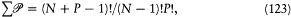Planck to Graetz, 23 Mar. 1897 (Deutsches Museum), quoted in Kuhn 1978, 265-266.

 Boltzmann 1877b.

63

where the sum is taken over all distributions (N0 , N 1 , . . . Ni , . . .) such that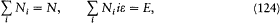and P is obtained by dividing the total energy E by e . It might be worth mentioning that this combinatorial formula was the one later used by Planck.

If the Ni 's are large enough to allow the Stirling approximation,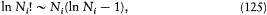W reaches its maximum value with the constraints (124) on the total number of particles and energy if for any i , and for Lagrange multipliers a and b .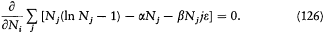This equation implies that N i must be proportional to e-ibe . Maxwell's distribution, or a discrete imitation of it, appears to be the "most probable" one, in the sense that it has the greatest number of complexions.

So much for the fiction. Boltzmann then turned to the more realistic continuous case. This was readily achieved by supposing that the energy unit e was small enough to consider that molecules whose energies lie between ie and (i + 1)e have the same energy. The numbers Ni now count the molecules in the various energy intervals. Provided that the sums over i can be approximated by integrals, the most probable number of molecules whose energy K lies within an infinitesimal energy interval dK is proportional to e-bKdK .

This is not yet Maxwell's law. To get it Boltzmann had to cut up the velocity space, instead of the energy axis, into uniform cells. Then Maxwell's expression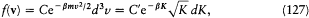was found to represent the most probable distribution of molecular velocities. Boltzmann also considered the positions r together with the velocities of the molecules. In this case the (r, v)-space has to be cut up into uniform cells, and Ni gives the number of molecules in the cell i . In the continuous limit the most probable distribution f (r, v) is uniform in the r-space and results in Maxwell's distribution in the velocity space. Furthermore, the logarithm of permutability may be calculated in this case to give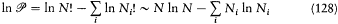64

or, in the continuous approximation,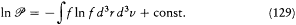In the case of maximal probability this expression of In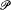, Boltzmann noted, is identical with the function -H and therefore gives the entropy of a perfect gas up to an additive constant (function of N ).

These calculations were simple enough, but their point of departure, the expression of the probability of a state distribution, needed further justification, as Boltzmann himself recognized: "I do not think that one is allowed to set this forth [that the equilibrium state is the most probable one] as something obvious, at least not without having first defined very precisely what is meant by the most probable state-distribution." He tried to justify the two main assumptions leading up to his expression for per-mutability, namely, the possibility of cutting up, and the uniformity of, (r, v)-space.

This uniformity, he said, resulted from the invariance of the differential element d3r d3v during a Hamiltonian evolution of a molecule. But the accompanying proof was either incomplete or wrong. In any case it could not fill the conceptual gap later emphasized by Einstein: a proper connection between the evolution in time of a system and the probability of its state was needed to justify not only uniformity in (r, v)-space but also, more generally, the relevance of combinatorial probabilities to thermodynamics.

Boltzmann's combinatorics, if not fully justifiable, had, at least, to be consistent. In this respect the recourse to finite cells could seem problematic. The number of molecules in a given cell had to be large (more precisely, there had to be a negligible number of cells for which Ni is neither zero nor very large) so that the Stirling approximation could be applied. At the same time the size of the cells had to be small enough that the sums over i could be approximated by integrals. Instead of directly investigating the consistency of these assumptions, Boltzmann preferred an analogy with familiar problems of kinetic theory:

Nevertheless, after closer inspection, this assumption must be regarded as obvious. Indeed, any application of differential calculus to gas theory rests on the same assumption. If for instance one wishes to calculate diffusion, viscosity, conductivity, etc., one has to admit in the same way that in every infinitesimal element of volume dx dy dz there is still an infinite number of gas molecules

 Ibid., 193.

 See Kuhn 1978, 55.

65

with velocity components lying between the limits u and u + du, v and v + dv, w and w + dw . This assumption means only that one can choose the limits for u, v, w , so that they include a very large number of molecules and that one may nonetheless regard all these molecules as having the same velocity components.

In the content of the 1877 memoir, one can easily check the legitimacy of this hypothesis for the most probable distribution of molecules. For instance, in the simplest case in which the energy axis is cut up into intervals of equal size e , the numbers Ni are given by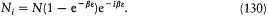It can easily be seen that the condition for the Stirling approximation to be valid is a large value of the number N0 of molecules in the zero-energy interval: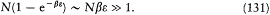The other condition, that the sums can be replaced with integrals, reads: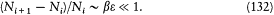The two conditions are both met ifConsequently, for any value of the available energy (E = N/b ) the size of the cells can be chosen consistently, and it then disappears from the final result for the most probable distribution and the corresponding entropy.

Since in Planck's later combinatorics e is not always a negligible fraction of 1/b ( = kT ) and appears in the final entropy formula, it is important to understand what makes it disappear in Boltzmann's case. The reason is not that e is infinitesimal in the mathematical sense; indeed, it must be larger than 1/Nb . At a purely formal level, the elimination occurs when sums like the one giving the entropy are replaced with integrals. Boltzmann had to take this formal step because the main physical quantity of interest, the distribution of molecules over cells (N0 , N 1 , . . . Ni , . . . ), was expected to be well approximated by a continuous distribution, the most probable of which is Maxwell's law. We will find that neither this circumstance nor its formal corollary occurs in Planck's combinatorics.

To summarize, Boltzmann's memoir of 1877 on entropy and probability was not very explicit about the physical meaning of its main procedural elements, the method of dividing up the space of configurations and the

 Boltzmann 1877b, 197-198.

66

uniformity of this space. It was clear to him, however, that combinatorial methods were relevant insofar as they were able to reproduce the "continuous" entropy formula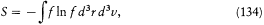which had already been founded on what he considered to be more fundamental bases, that is, on the ergodic hypothesis or on the methods of the H -theorem. On the contrary, Planck, still unfamiliar with the foundations of Boltzmann's theory, would venture to confer physical meaning on the artifact of energy elements.

### Quantified Chaos

When in late 1900 Planck tried to apply Boltzmann's combinatorial method to his resonator, he naturally drew his inspiration from the marked analogy between the H -theorem for gases and that for radiation. The pivot of this analogy had to be the principle of disorder, since it was at the center of his conception of irreversibility. In this regard a very typical statement of Planck reads, "One can speak of a disorder, therefore of an entropy of a resonator" (March 1900). As we saw, Planck identified the kind of disorder affecting a resonator as the unobservable temporal fluctuation of the energy Y(t) of this resonator around its secular average U . More generally, disorder was what made the microscopic details of the description of a system irrelevant to the evolution of the really accessible parameters of the system, namely spatial (in the gas case) or secular (in the radiation case) averages.

In harmony with his idea of the centrality of disorder, Planck perceived Boltzmann's permutability as a quantitative measure of molecular chaos, whereas Boltzmann never tried to make such a direct connection between entropy and disorder. For a gas disorder is what makes the detailed microscopic configuration irrelevant to the evolution of the distribution f (v) or f (r, v) entering the Boltzmann equation. Permutability, being a measure of the number of microscopic configurations compatible with the distribution f , appeared to Planck as a natural quantitative measure of this disorder. In the resonator case, the counterpart of the permutability had to be something like the number of functions Y(t) compatible with a given average energy U , since the disorder lay in the uncontrollable irregularity of the instantaneous energy.

 Planck 1900b, PAV 1:674.

67

There is no doubt that this relation between entropy and disorder was the key point of Planck's published reasoning. His famous communication of 14 December 1900 to the Berlin Academy introduced the new determination of the entropy of a resonator with the words "Entropie bedingt Unordnung," that is, "Entropy presumes disorder." It continued with a description of the nature of the disorder of a resonator drawn from his previous theory that justified his subsequent computation of the number of complexions, or "probability," leading to the entropy of a resonator. Planck's use of the word "probability" in this context should not confuse the reader: he just meant it in the mathematical sense of an abstract lottery game leading to combinatorial expressions.

Admittedly, Planck's presentation did not necessarily reflect the way he really reached his derivation of the blackbody law. It would seem plausible that he worked backward from the radiation law and guessed the proper combinatorics from the form of the resonator entropy (as in Rosenfeld's reconstruction, for instance). According to a letter from Planck to Lummer of 26 October 1900, the truth seems to have lain somewhere in between; that is to say, Planck simultaneously used inductive (from the blackbody law to entropy) and deductive (from entropy to the blackbody law) considerations:

If the prospect should exist at all of a theoretical derivation of the radiation law, which I naturally assume, then, in my opinion, this can be the case only if it is possible to derive the expression for the probability of a radiation state, and this, you see, is given by the entropy. Probability presumes disorder, and in the theory I have developed, this disorder occurs in the irregularity with which the phase of the oscillation changes even in the most homogeneous light. A resonator, which corresponds to a monochromatic radiation, in resonant oscillation will likewise show irregular changes of its phase [and also of its instantaneous energy, which was more important to Planck's subsequent derivation], and on this the concept and the magnitude of its entropy are based. According to my formula [the blackbody law communicated on 19 October to the German Academy], the entropy of the resonator should come to: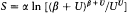[formula (119)], and this form very much recalls expressions occurring in the probability calculus. After all, in the thermodynamics of gases, too, the entropy S is the log of a probability magnitude, and Boltzmann has already stressed the close relationship of the function X x , which enters the theory of combinatorics, with the thermodynamic entropy. I believe, therefore, that the prospect would certainly exist of arriving at my formula by a theoretical route, which

 Planck 1900d.

68

would then also give us the physical significance of the constants C and c [of Planck's law].

In any case, the final justification of Planck's calculation in terms of quantified chaos certainly determined his opinion about the status of the finite energy elements.

Having described the nature of the disorder to be found in a resonator, Planck continued his communication as follows. In order to give a definite meaning to the number W of evolutions Y(t) of the energy compatible with a given temporal average U , he replaced Y(t) with its value at N different instants of time, or, more exactly, with the energy values of N independent (far removed from one another) identical resonators at one given instant. Then W is represented by the number of distributions of a total energy E = NU over these N resonators.

For the rest, Planck proceeded in exact analogy with Boltzmann's "fiction." That is to say, he divided the energy E into finite elements e :

If E is taken to be an indefinitely divisible quantity, the distribution is possible in an infinite number of ways. But I regard E —and this is the essential point of the whole calculation—as made up of a completely determinate number of finite equal parts, and for this purpose I use the constant of nature h = 6.55 × 10-27 (erg · sec). This constant, once multiplied by the common frequency of the resonators, gives the energy element e in ergs, and by division of E by e we get the number P of energy elements to be distributed over the N resonators. When this quotient is not an integer, P is taken to be a neighboring integer.

According to this hypothesis, W becomes the total number of complexions compatible with the total energy E , wherein the word "complexion" is defined strictly in Boltzmann's sense, by attributing to each resonator a given discrete energy (as specified in (121)). One could calculate this number by adding the permutabilities of all the distributions (N0 , N 1 , . . .,Ni , . . .) (in Boltzmann's notation) such that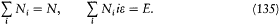For the sake of simplicity, however, Planck preferred to compute W directly as the "number of distributions of P energy elements over N resonators," it being understood that only the number (not the identity) of the energy

 Planck to Lummer, 26 Oct. 1900, quoted in Jungnickel and McCormmach 1986, 2: 261-262.

 Planck's W stands for Wahrscheinlichkeit (probability).

 Planck 1900d, PAV 1:700-701.

 N can always be chosen so great that the constraint does not play any role. In Planck 1906, 151, the characterization of W as a sum of permutabilities comes first; then Planck switches to the "faster and easier" method of the energy elements.

69

elements attributed to a given resonator is considered. The latter stipulation surprised some of Planck's readers (Ehrenfest and Natanson), but it was in fact implied by the analogy with Boltzmann's fiction.

For the W formula Planck referred his reader to the calculus of combinations. Here follows an elegant proof, due to Ehrenfest and Kamerlingh Onnes (1914). A complexion may be represented as a symbol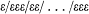containing P times the symbol e and N - 1 times the symbol/. The number of complexions is therefore equal to the number (N + P - 1)! of all these symbols regarded as different, divided by the number, P !, of permutations of the e symbols and by the number, (N - 1)!, of permutations of the / symbols: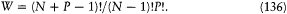Adapting Boltzmann's relation between entropy and probability to this problem, Planck wrote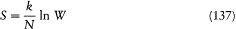for the entropy S of a single resonator. The constant k , Planck emphasized, had to be the same in gas theory and in radiation theory (Boltzmann did not need such a constant, since he measured temperatures in energy units). But contrary to Boltzmann's case, no procedure of extremum was here necessary: as a consequence of the different type of disorder, the average energy U , not the more detailed distribution (N0 , N 1 , . . . Ni , . . .), characterizes the "macroscopic" state of the resonators.

As N , the number of exemplars of the resonator, can be taken as great as one wishes, the Stirling approximation applies: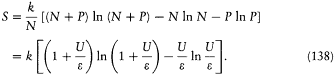On Ehrenfest's and Natanson's reactions and Planck's reply, see Darrigol 1988b, 52.

 Ehrenfest and Kamerlingh Onnes 1915.

70

From the relation between entropy and temperature, 1/T = dS/dU , results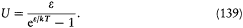The fundamental relation (96) and e = hv finally give: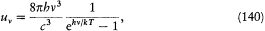which is the canonical form of Planck's law.

In a subsequent publication Planck explained that the proportionality of the energy element e to the frequency was implied by Wien's displacement law (expressed in the form (98)). Later, in 1906, he showed that this property and also the uniformity of the cutting up of the energy axis resulted from Boltzmann's general assumption of uniformity in configuration space (here the (f , Lf[*] )-plane) and from the quadratic form of the energy of a resonator. These remarks made the analogy with Boltzmann's combinatorics even closer.

### Quantum Continuity

Table 2 summarizes the formal correspondence between Boltzmann's "fiction" and Planck's combinatorics of N exemplars of a resonator.

Despite the exact transposition of the definition of a complexion, this correspondence is certainly not the most direct that one could imagine. Had he not been guided by his interpretation of W as a measure of disorder, Planck would no doubt have characterized the macrostate by a distribution (N0 , Ni , . . . Ni , . . .), in the resonator case as in the gas case. This would have led to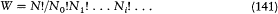and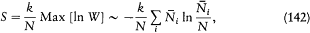71
 TABLE TWO ANALOGY BETWEEN PLANCK'S AND BOLTZMANN'S COMBINATORICS Gas Resonators Microstate Complexion: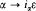Complexion:Macrostate Energy partition: (N0 , N 1 , . . ., N1 ,.. ) Total energy: E = NU Number of complexions Permutability: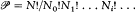"Probability" W = (N + P - 1)!/(N - 1)!P ! "Boltzmann's principle"S = k In W Uniformity in (r, v)-space in (f, Lf[*] )-plane

where the distribution (N0 , N1 , . . ., Ni , . . .) is the one for which W reaches its maximum, with the constraints (135)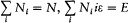An elementary calculation by the method of Lagrange multipliers (see equation 126) gives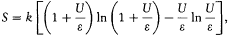which is identical with Planck's expression (138) (this identity results from the fact that most complexions belong to distributions that are very close to the most probable one).

However, if the distributions (N 0 , N1 , . . ., Ni , . . .) really played similar roles in the gas case and in the resonator case, they would have to be replaced in both cases by their continuous limits, formally obtained by setting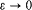in the above expressions. The expression (139) for the resonator energy would therefore become U = kT , and instead of Planck's law one would have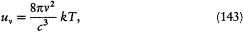The above calculation provided the formal basis for the derivation of Planck's law first given in Lorentz 1910 (see Darrigol 1988b, 62-63) and interpreted in terms of an intrinsic discontinuity of the resonator energy.

72

a result incompatible with experiments, and even absurd since it leads to an infinite energy for the entire spectrum.

Because his conception of disorder implied a combinatorics different from Boltzmann's, Planck avoided this catastrophic conclusion. However, a deeper understanding of the foundations of Boltzmann's theory would have left him no choice. As Einstein correctly pointed out in 1905, Planck's resonators could be brought to interact with the molecules of a gas, without their interaction with radiation being substantially modified. In such a system, the energy distribution of the resonators and the velocity distribution of the molecules play parallel roles. Consequently, the reasoning just given should apply, and the formula U = kT should hold, as a particular case of energy equipartition.

In conformity with the original object of this program, Planck reasoned purely in terms of radiation theory and did not consider such an admixture of molecular and electrodynamic systems. Furthermore, like most of his colleagues, he did not believe in the generality of the equipartition law, from which the relation U = kT trivially resulted. This law generally led to much too high values of specific heats of materials, and the best specialists, including Lord Kelvin and Boltzmann (with the exceptions of Gibbs and Maxwell, who died prematurely), attributed this failure to some unknown intricacies of molecular dynamics.

Planck's only guide was his characterization of disorder in radiation theory, which deprived the energy distribution (N0 , N1 , . . . Ni , . . .) of a direct physical meaning and made the secular energy U the only observable property of resonators (except for their frequency, of course). The energy elements had therefore no reason to disappear from the end results. Being the gauge of elementary disorder, they fitted harmoniously, like the hypothesis of natural radiation, in the logical "gap" open because of the indetermination of the detailed structure of resonators; they started to play a role where ordinary electrodynamics ceased to provide definite information. In other words, electrodynamic laws and Planck energy elements did not contradict each other, they complemented each other.

In this context Planck could not possibly have understood the introduction of energy elements as a discrete selection of the admissible energy

 This result is called the "Rayleigh-Jeans" law. It was first obtained by Rayleigh in 1900, up to a numerical mistake corrected by Jeans in 1905. The original derivation rested on an application of the equipartition theorem to the stationary modes of a cavity. However, Rayleigh and Jeans did not believe in the validity of this theorem for large frequencies. See Kuhn 1978.

 Einstein 1905.

 See Brush 1976, 356-363, and Kuhn 1978.

73

values of a resonator. Such a discontinuity would have contradicted the rest of his theory, especially the proof of the fundamental equation (96), which entered the derivation of the blackbody law. Moreover, Planck's own wording of the "essential point" of his communication of 14 December leaves no room for doubt. Immediately after introducing the energy elements, he wrote: "When this quotient [E/e ] is not an integer, P is taken to be a neighboring integer." This by itself shows that the energy of N independent resonators, and a fortiori the energy of a single resonator, was not thought to be restricted to multiples of e

To be the counterpart of Boltzmann's "fiction," Planck's discrete complexions also had to be fictitious. This point was made entirely explicit in the lectures on the theory of thermal radiation of 1905-6: a complexion, Planck said, signified the attribution to each resonator of an "energy domain" delimited by the energy values ie and (i + 1)e , not of a discrete energy value ie . In conformity with this viewpoint, the fundamental notion of Planck's subsequent theory of quantization was that of "elementary domains of probability," a generalization of the energy domains in configuration space. This conception stood against the notion of discrete quantum state introduced by Einstein, raised to a fundamental postulate by Niels Bohr and adopted by most early quantum theorists.

### Summary and Conclusions

The Berlin spectroscopists did not let Planck rejoice for long about his fundamental derivation of Wien's law. In the very year Planck completed his program, 1899, they began to observe systematic deviations from Wien's law in the infrared part of the blackbody spectrum. This helped Planck realize that, contrary to his earlier conviction, there were an infinite number of expressions for the resonator entropy compatible with his electromagnetic H -theorem, and thus an infinity of corresponding blackbody laws. In fact, in order for the total entropy to increase, the only constraint on the expression for the resonator entropy was that its second derivative (with respect to energy) should be negative. Then, on the basis of a new independent argument, Planck imposed an additional constraint on this derivative and recovered Wien's law, to the experimenters' great disbelief.

 See n. 90. The absence of a quantum discontinuity in Planck's early derivations of his blackbody law was first observed by Kuhn 1978 against a long historical tradition that asserted the contrary.

 Planck 1906, 135: ". . . resonators that carry a given amount of energy (better: that fall into a given 'energy domain') . . ." The notion of Elementargebiete der Wahrscheinlichkeit was systematically developed in the second edition (1913) of Planck 1906.

74

The new argument was wrong, and Planck publicly withdrew it in October 1900, after the experimental violations of Wien's law had become more obvious. In the same communication he proposed an alternative blackbody law, a happy guess based on a simple modification of the expression for the second derivative of the resonator entropy corresponding to Wien's law. The new blackbody law immediately proved to fit empirical data quite well, and Planck started to think about a more fundamental derivation. This led him to consider the relation between entropy and probability which Boltzmann had introduced in 1877.

According to the relevant memoir of Boltzmann, in a dilute gas the equilibrium distribution of velocities—that is, Maxwell's distribution—was also the most "probable"; and the entropy (or the function -H ) was given by the logarithm of the (unnormalized) "probability." Calling (according to modern terminology) the exact microscopic configuration of the molecular model a microstate, and the distribution of velocities a macrostate, Boltzmann's (unnormalized) "probability" was defined as the number of microstates compatible with a given macrostate. Of course, this definition has problems since there is a continuous infinity of microstates corresponding to every macrostate. To solve this difficulty, Boltzmann divided up the configuration space of a molecule into cells and regarded all configurations belonging to a given cell as one single configuration. For instance, in a simple model for which the configuration of a molecule is completely determined by its energy, the energy axis is cut up into equal intervals or energy elements, and a microstate is obtained by assigning to each molecule one of these intervals. Boltzmann's subsequent calculations required the energy elements to be finite (so that the number of molecules in an energy interval could be very large) but small enough not to blur the definition of macrostates, to which the quantities of physical importance pertained. On this condition the energy elements disappeared from the end results; and Maxwell's distribution and the corresponding entropy were recovered. In other words, Boltzmann employed the energy elements as a mathematical artifice, for the purpose of giving a definite meaning to the "probability" of a macrostate. They did not belong to the microscopic model, nor could they enter macroscopic laws; for these could be reached independently of the relation between entropy and "probability," through the H -theorem or the ergodic hypothesis.

The relation between entropy and probability played only a minor role in Boltzmann's subsequent work. For instance, in his Gastheorie it appeared only as a "mathematical illustration" of the expression for the H -function. Boltzmann (rightly) believed that derivations of thermodynamic

75

quantities and laws through the H -theorem or through the ensemble technique were more fundamental. In 1900 Planck faced a different situation: his electromagnetic H -theorem had proved useless in determining the entropy of a resonator, so that the relation between entropy and probability, far from being superfluous, seemed to be the only available access to the blackbody law. Planck accepted the relation but not its original context, which was a probabilistic interpretation of the irreversibility theorem. Instead he reinterpreted Boltzmann's "probability" as a quantitative measure of elementary disorder, a notion that was at the core of his (Planck's) non-probabilistic conception of irreversibility. Such reinterpretation also had a practical advantage: it provided some guidance about how to extend the analogy between gas theory and radiation theory.

Planck first discussed the type of disorder to be found in a resonator, knowledge gleaned from the requirements of derivation of the electromagnetic H -theorem. In this way he determined what played the roles of microstates and macrostates, as the states of the system respectively in the detailed and the physical levels of description. Next, following Boltzmann, he introduced finite energy elements in order to obtain a definite value for the "probability," that is, the number of microstates in a given macro-state. The logarithm of this "probability" gave him the entropy of a resonator, which leads to Planck's new blackbody law—if only the energy elements can be taken to be proportional to the frequency of the resonator.

Contrary to Boltzmann's case the energy elements now appeared in the final thermodynamic expressions. Planck attributed this peculiarity to a difference in the type of disorder. Indeed, his understanding of the disorder in a resonator led to a notion of macrostate (characterized by the total energy of an ensemble of resonators) that was insensitive to the introduction of energy elements; therefore, Boltzmann's condition that the energy elements should be small enough not to blur the definition of macrostates had no counterpart in Planck's case, and nothing seemed to forbid the appearance of the energy element in the final entropy formula.

In this situation Planck had no reason to question the continuity of the resonator energy. Moreover, such a step would have contradicted, among other things, his derivation of the "fundamental equation," which was necessary for his proof of the blackbody law. In his mind the energy elements were something like the gauge of elementary disorder; they therefore pertained to the indeterminate internal structure of resonators, and they did not contradict his electrodynamic reasonings, which were independent of this structure. In short, Planck relaxed Boltzmann's connection between microworld and macroworld by leaving part of the micromodel

76

indeterminate. This allowed him to maintain strict irreversibility in the macroworld, by adjusting the indeterminate part of the micromodel (introduction of elementary disorder). In turn, this adjustment permitted his derivation of the blackbody law, without contradicting the determinate part of the micromodel.

As is well known, a few years ago Thomas Kuhn published an in-depth study of blackbody theory at the turn of the century. I will briefly indicate how my account may differ from his. Kuhn concludes, as I do, that Planck did not restrict the energy of his resonators to discontinuous values. His reasoning may be summarized as follows: Boltzmann introduced finite energy elements with no intention of jettisoning the continuity of molecular dynamics; Planck reached his expression for the resonator entropy working in close analogy with Boltzmann's method; therefore, despite some delusive formal manipulations, he did not quantize the energy of the resonators. As convincing as it might be, this argument does not say why Planck did not feel compelled, within the framework of his own thermodynamics, to imitate Boltzmann's procedure even more closely, which would have led to an absurd blackbody law (the so-called Rayleigh-Jeans law). My explanation for this rests on the idiosyncratic nature of Planck's conception of the microscopic foundations of thermodynamics. Kuhn describes Planck's conversion to Boltzmann's views and methods as quasi-complete (as starting with the introduction of "natural radiation"). In fact, as Allan Needell first demonstrated, Planck did not renounce his nonstatistical conception of irreversibility until much later (around 1914). This in turn explains the role elementary disorder played in orienting Planck's use of analogies in his derivation of the blackbody law in 1900. It also explains why Planck's early readers (and a good number of later ones) found his derivation either obscure or implicitly based on an intrinsic quantization of resonators: they were wearing Boltzmann's spectacles.

During the first ten years of this century, Planck's theory of radiation, and more generally the problem of thermal radiation, became the object of critical investigations by unusually penetrating minds, among whom were two young physicists, Ehrenfest and Einstein, and the venerated H. A. Lorentz. Some of Planck's results survived: the electromagnetic H -theorem (so named by Ehrenfest) proving the spatial uniformizing effect of resonators, and the blackbody law with its characteristic energy elements and the new fundamental constant h .

 Kuhn 1978; Needell 1980, 1988.

 See Kuhn 1978; Klein 1963b, 1967; Darrigol 1988b.

77

However, the central concept of Planck's theory, namely his notion of elementary chaos, appeared to be untenable. According to Einstein, no coherent conception of microscopic dynamics was able to provide a strict and indefinite increase of entropy. On the contrary, microscopic disorder implied observable effects like the perpetual agitation of Brownian particles and mirrors. Within Boltzmannian orthodoxy, Planck's assumption of finite energy elements proved to be incompatible with the foundation of electrodynamics. No interpretation of the blackbody law could be given without emancipating the resonators from their classical (even secular) behavior.

In 1906 Einstein reinterpreted the formal skeleton of Planck's derivation of the blackbody law on the basis of a discrete quantization of resonators. In other words, he turned Boltzmann's "fiction" into a reality, interpreting the energy unit as the minimal amount of energy that resonators could exchange with radiation. This idea of a radical quantum discontinuity was certainly paradoxical, for no one (not even Einstein) could imagine a satisfactory mechanism of the quantum jumps. Nevertheless, it quickly led Einstein to a successful theory of specific heat. By the Solvay congress of 1911 an increasing number of specialists (but not Planck) were convinced that the energy of atomic entities could only take discrete values. The ground was ready for even sharper departures from classical theory, which Bohr soon brought with his atomic theory.

To conclude, the retrospective successes and defects of Planck's program can be largely understood as deriving from certain powerful analogies with Boltzmann's theory, these analogies being constrained by a belief in the absolute validity of the entropy principle (which was not Boltzmann's). One of these successes, the electromagnetic H -theorem, depended upon a formal analogy between the notions of natural radiation and of molecular chaos. Further, the conception of disorder bound to this analogy guided Planck in his exploitation of another analogy, that between Boltzmann's combinatorics and resonator combinatorics. The resulting derivation of the blackbody law happened to be formally meaningful, even though its conservative interpretation would not survive the quantum revolution initiated by Einstein.

 Einstein 1906. See Kuhn 1978, and Klein 1965.

79Chapter IV The Infrared ChallengePreferred Citation: Darrigol, Olivier. From c-Numbers to q-Numbers: The Classical Analogy in the History of Quantum Theory. Berkeley:  University of California Press,  1992. http://ark.cdlib.org/ark:/13030/ft4t1nb2gv/